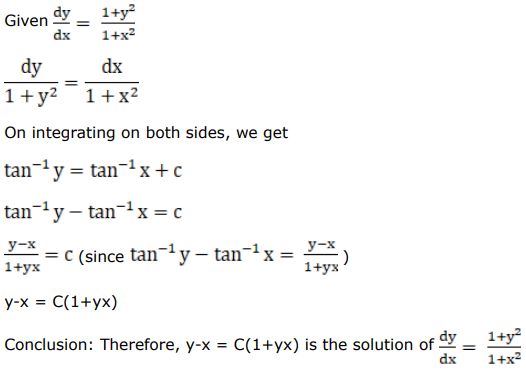# Mark against the correct answer in the following:

Question:

Mark $(\sqrt{)}$ against the correct answer in the following:

The solution of the $\operatorname{DE} \frac{d y}{d x}=\frac{\left(1+y^{2}\right)}{\left(1+x^{2}\right)}$ is.

A. $(y+x)=\mathrm{C}(1-y x)$

B. $(y-x)=\mathrm{C}(1+y x)$

C. $y=(1+x) C$

D. None of these

Solution: